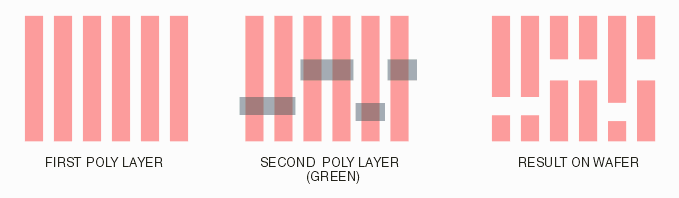## Derived Layers using Boolean Operations

Many of the newer layout files will require a Boolean operation on more than one layer in order to enable correct net tracing. This is due to advanced lithography approaches that start with an array of parallel stripes. A second layer then "cuts" the stripes where needed.The net tracing is done on the "derived" layer - not on either of the actual GDSII layers.

The client controls this behavior by defining it in the stackup file.

### Derived Layer Specification

So how do we instruct Nettrace to produce and use a "derived" layer. There are actually two steps: a) generate a new derived layer by defining operations on existing layers; b) use the "derived" layer in the conductor (METAL) stackup or in other sections.

1. A derived layer number must be represented as 00N (N > 0) and 00N uniquely identifies a single derived layer. e.g 001, 0031 etc.

2. Every derived layer MUST be represented by a '00' number and the presence of a '00' number in any of the sections of the technology (.tlib) file will be interpreted as a derived layer.

3. A derived layer can be used anywhere a standard layer & datatype (L:D) or a set of layers with the same layer number (L) can be used in the technology file.

4. All derived layers MUST have a definition.

Defining a Derived Layer

A derived layer is defined inside of a block known as a SYNTHESIZE block.

```SYNTHESIZE
#Derived_Layer_Tag    Operation
0017                  17:20 - 17:30
ENDSYNTHESIZE
```

In the example above, a derived layer called 0017 is "built" by subtracting the data found on 17:20 from the data found on 17:30. This might be done if boundaries on 17:20 represented the vertical stripes used for poly and boundaries on 17:30 represented boundaries cut away from those stripes.

If one needs to generate multiple derived layers, additional lines can be entered into a single SYNTHESIZE block as shown below:

```SYNTHESIZE
#Derived_Layer_Tag    Operation
0017                  17:20 - 17:30
0020                  20 | 120 | 122 | 124
ENDSYNTHESIZE
```

Operations

Available operations between layers are:

' + ' (BINARY UNION)

' - ' (DIFFERENCE)

' & ' (INTERSECTION)

' ^ ' (XOR)

' | ' (AGGREGATION/ASSIGNMENT)